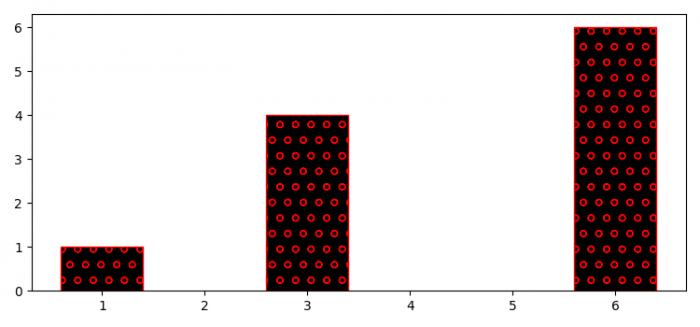# How to decouple hatch and edge color in Matplotlib?

MatplotlibServer Side ProgrammingProgramming

To decouple hatch and edge color in matplotlib, we can use hatch color “o” and edge color “red”.−

## Steps

• Create a new figure or activate existing figure.
• Add a subplot arrangement to the current axes.
• Create two lists of data points.
• Use bar() method with hatch and edgecolor.
• To display the figure, use show() method.

## Example

import matplotlib.pyplot as plt
plt.rcParams["figure.figsize"] = [7.50, 3.50]
plt.rcParams["figure.autolayout"] = True
fig = plt.figure()
plt.show()